Field codes: Eq (Equation) field

from Microsoft Word 2003 Help

Some of the content in this topic may not be applicable to some languages.

{ EQ Instructions }

Produces a mathematical equation. Equation Editor is the recommended tool for creating equations. However, you can use the EQ field if you have not installed Equation Editor or if you want to write inline equations. An EQ field cannot be unlinked. If you double-click an EQ field, Microsoft Word converts the field to an embedded Equation Editor object.

Security  Because field codes can be visible to anyone reading your document, be sure that the information you place in field codes is not information that you want kept private.

Switches specify how to build the equation with the elements enclosed in parentheses. You can modify a switch by using the appropriate switch options.

Notes

• To use a comma, open parenthesis, or backslash character in a resulting equation, precede the symbol with a backslash: \, \( \\.
• Some switches require a list of elements separated by commas or semicolons. Use commas as the separators if the decimal symbol for your system is a period (specified as part of the regional settings in Microsoft Windows Control Panel). If the decimal symbol for your system is a comma, use semicolons.

Switches

Array switch: \a()

Arrays elements in multiple columns; elements appear in order by rows. The following options modify the \a switch.

\al

Aligns left within columns.

\ac

Aligns center within columns.

\ar

Aligns right within columns.

\con

Arrays elements in n columns (the default is 1).

\vsn

Adds n points of vertical spacing between lines.

\hsn

Adds n points of horizontal spacing between columns.

Example

{ EQ \a \al \co2 \vs3 \hs3(Axy,Bxy,A,B) } displays the following:

 Axy Bxy A B

Bracket: \b()

Brackets a single element in a size appropriate to the element. The default brackets are parentheses. The following options can modify the \b switch.

\lc\c

Draws the left bracket using the character c.

\rc\c

Draws the right bracket using the character c.

\bc\c

Draws both bracket characters using the character specified for c.

If the character you specify is {, [, (, or <, Word uses the corresponding closing character as the right bracket. If you specify any other character, Word uses that character for both brackets.

Example

{ EQ \b \bc\{ (\r(3,x)) } displays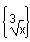.

Displace: \d()

Controls where the next character following the EQ field is drawn. The following options can modify the \d switch. Note that empty parentheses follow only the last option in the instructions.

\fon ()

Draws to the right n points.

\ban ()

Draws to the left n points.

\li ()

Underlines the space up to the next character.

Example

{ EQ \d \fo10 \li() } displays the following:

(preceding text) __ (following text)

Fraction: \f(,)

Creates a fraction with the numerator and denominator centered above and below the division line, respectively. If your system uses a comma as the decimal symbol, separate the two elements with a semicolon (;).

Example

{ EQ \f(2,RateChange) } displays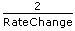.

Integral: \i(,,)

Creates an integral, using the specified symbol or default symbol and three elements. The first element is the lower limit, the second is the upper limit, and the third is the integrand. You can use the following options to modify the \i switch.

\su

Changes the symbol to a capital sigma and creates a summation.

\pr

Changes the symbol to a capital pi and creates a product.

\in

Creates the inline format with the limits displayed to the right of the symbol instead of above and below it.

\fc\c

Substitutes a fixed-height character specified by c for the symbol.

\vc\c

Substitutes a variable-height character specified by c for the symbol. The symbol matches the height of the third element.

Example

{ EQ \i \su(1,5,3) } displays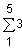.

List: \l()

Uses any number of elements to create a list of values separated by commas or semicolons, so that you can specify multiple elements as a single element.

Example

{ EQ \l(A,B,C,D,E) } displays A,B,C,D,E.

Overstrike: \o()

Places each successive element on top of the previous one. Any number of elements is permitted. Separate multiple elements with commas.

Each character is printed within an invisible character box. Options align the boxes on top of one another. You can use the following options to modify the \o switch.

\al

At the left edge.

\ac

In the center (the default).

\ar

At the right edge.

Draws a radical using one or two elements.

Example

{ EQ \r(3,x) } displays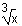.

Superscript or Subscript: \s()

Places elements as superscript or subscript characters. Each \s code can have one or more elements; separate the elements with commas. If more than one element is specified, the elements are stacked and left-aligned. You can use the following options to place single elements after the \s switch.

\ain ()

Adds space above a line in a paragraph by the number of points specified by n.

\upn ()

Moves a single element above the adjacent text by the number of points specified by n. The default is 2 points.

\din ()

Adds space below a line in a paragraph by the number of points specified by n.

\don ()

Moves a single element below the adjacent text by the number of points specified by n. The default is 2 points.

Example

{ EQ \s\up8(UB)\s\do8(2) } displays:

UB2 (Regular text)

Box: \x()

Creates a border for an element. When used without options, this code draws a box around the element. You can combine the following options to modify the \x switch.

\to

Draws a border above the element.

\bo

Draws a border below the element

\le

Draws a border to the left of the element.

\ri

Draws a border to the right of the element.

Example

{ EQ \x \to \bo(5) } displays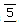.